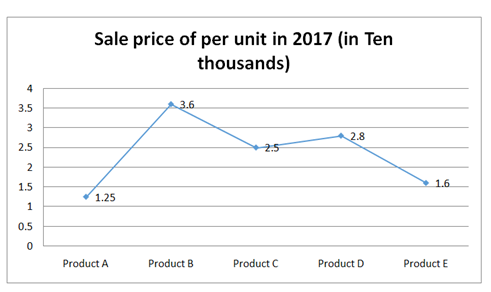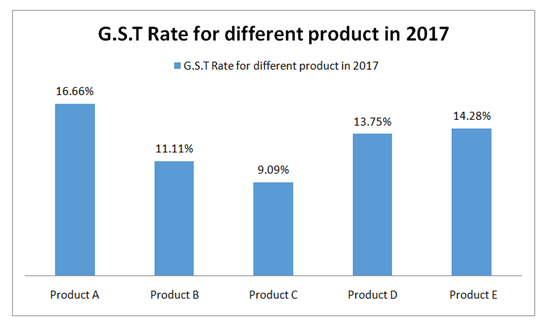# IBPS Clerk Prelims Quantitative Aptitude Questions 2019 (Day-09)

Dear Aspirants, Our IBPS Guide team is providing new series of Quantitative Aptitude Questions for IBPS Clerk Prelims 2019 so the aspirants can practice it on a daily basis. These questions are framed by our skilled experts after understanding your needs thoroughly. Aspirants can practice these new series questions daily to familiarize with the exact exam pattern and make your preparation effective.

Check here for IBPS Clerk Prelims Mock Test 2019

[WpProQuiz 7308]

Data Interpretation

Directions (1 – 5): Study the following information carefully and answer the questions given below.Note: GST charged on total sale.

1) If the company had manufactured 12000 units of product A in 2017. Then find the total GST paid by company in 2017 on product A.

a) 3 cr

b) 2.5 cr

c) 3.2 cr

d) 2.8 cr

e) None of these

2) If the company had paid the total GST of Rs 3 cr on the product C in 2017. Find the total number of Product C manufactured by Company in 2017.

a) 13200

b) 12500

c) 13000

d) 11700

e) 12000

3) If the company had done the tax evasion on product B in 2017 and paid 12.5% less than the actual GST. Find the amount paid by company for 18000 units of product B as GST after tax evasion in 2017.

a) 8.2 cr

b) 6.3 cr

c) 5.5 cr

d) 6.7 cr

e) None of these

4) If company had manufactured 16000 units of product D and 28000 units of product E in 2017. Then GST paid company D is how much percent less than GST paid by Company E in 2017.

a) 3 %

b) 3.90%

c) 3.75%

d) 5%

e) None of these

5) If in 2018 price per unit of product D increases by 20% then find the difference in GST paid by company from previous year. If company had made equal units of products C in both years.

a) Rs 42000

b) Rs 44000

c) Rs 22000

d) Rs 24000

e) Cannot be determined

Application Sums

6) An amount  x is invested in scheme A for 2 years at 10% p.a compounded annually and an amount y is invested in scheme B for 3 years at 16% p.a simple interest. If the interest earned by scheme A is 87.5% of interest earned by scheme B and the total sum of amount invested in both scheme is Rs 9000. Then find the amount invested in scheme A.

a) 6000

b) 3000

c) 4500

d) 5500

e) None of these

7) B alone can do a piece of work in 25 days and A is 16.66% inefficient less than B. A and B started working together for 6 days then C alone completed the rest work in 21 days. In how many days C alone can complete the whole work?

a) 37.5 days

b) 38 days

c) 42 days

d) 45 days

e) None of these

8) A bag contains x green balls, 4 red balls and 5 white balls 3 balls are picked up randomly one after the another without replacement from the bag. Find the value of x if probability of 3 balls being green is 1/16.

a) 7

b) 6

c) 8

d) 9

e) None of these

9) A purchased an old scooter for Rs 30000 and spent 3500 in servicing, 1500 in engine repair. If he wants to earn 20% profit then at what price he must have to sell the scooter?

a) Rs 45000

b) Rs 42000

c) Rs 32000

d) Rs 50000

e) None of these

10) A and B started a business by investing Rs 2500 and Rs 2200 respectively. After 7 months they had invested 500 and 800 more amounts respectively. If the difference between the share of A and B is Rs 3486, find the total share earned after 1 year.

a) Rs 101152

b) Rs 104452

c) Rs 104414

d) Rs 102369

e) None of these

Directions (1-5) :

Number of units = 12000

Price per units = 12500

Sale = 12000 * 12500 = 15 cr

Total GST paid = 16.66% of 15 cr = 1/6 * 15  = 2.5 cr

Let the total number of product C manufactured by company = x units

Price per units = 25000

Sale = 25000x

According to the question,

25000x * 9.09% = 3 cr

x = 30000000/25000 * 11 = 13200  (9.09% = 1/11)

Number of units = 18000

Price per unit = 36000

Total sale = 18000 * 36000 = 64.8 cr

Actual GST = 11.11% of 64.8 cr = 648000000 * 1/9 = 7.2 cr    (11.11% = 1/9)

Tax evasion  = 12.5% of 7.2 cr = 1/8 * 72000000 = 90 lakhs

Required amount = 7.2 – 0.90 = 6.3 cr

Number of units of product D = 16000

Price per unit = 28000

Sale = 16000 * 28000 = 44.8 cr

GST = 13.75% of 44.8 cr = 13.75/100 * 44.8 cr = 6.16 cr

Number of units of product E = 28000

Price per unit = 16000

Sale = 28000 * 16000 = 44.8 cr

GST = 14.28 % of 44.8 cr = 1/7 * 44.8 cr = 6.4 cr

Required % = (6.4 – 6.16)/6.4 * 100 = 3.75%

We don’t know the information about the number of units manufactured in 2017 and 2018. Hence, the answer of the question cannot be determined.

Directions (6-10) :

x + y = 9000 ——– i

In scheme A,

Net effective rate = 10 + 10 + (10 * 10)/100 = 21%

Interest = 21x/100

Net effective rate = 3 * 16 = 48%

Interest = 48y/100

According to question,

48y/100 * 87.5/100 = 21x/100

48y/100 * 87.5 = 21x

4200y/100 = 21x

2y = x —— ii

Putting (ii) in (i)

x + y = 9000

2y + y = 9000

y = 3000

x = 9000 – 3000 = 6000

Time taken by B alone = 25 days

Ratio of efficiency of A and B = 5 : 6

Ratio of time taken by A and B = 6 : 5

Therefore, time taken by A alone = 30 days

Total work = LCM of 30 and 25 = 150 units

1 day work of A = 150/30 = 5 units

1 day work of B = 150/25 = 6 units

1 day work of (A + B) = 5 + 6 = 11 units

6 days work of (A + B) = 7 * 11 = 66 units

Left work = 150 – 66 = 84 units

Now, C can complete 84 units = 21 days

150 units = 21 * 150/84 = 37.5 days.

Probability of getting 1st green ball = x/(x + 4 + 5) = x/x + 9

Probability of getting 2nd green ball without replacement = (x – 1)/(x + 8)

Probability of getting 3rd green ball without replacement = (x – 2)/(x + 7)

According to the question,

[x/x + 9] * [(x – 1)/(x + 8)] * [(x – 2)/(x + 7)] = 1/16

Now we will check through option and hence, option A = 7 satisfied the equation

So, value of x = 7

Total C.P of scooter = (30000 + 3500 + 1500) = Rs 35000

Required Profit = 20%

So, required S.P = 120/100 * 35000 = Rs 42000

Ratio of shares of A and B = (2500 * 7 + 3000 * 5) + (2200 * 7 + 3000 * 5)

= 325: 304

Let the total profit earned after 1 year = x

So, (325 – 304)/(325 + 304) * x = 3486

21x/629 = 3486

x = Rs 104414